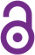# Chrestenson transform FPGA embedded factorizations

Michael J. Corinthios

Article (2016)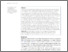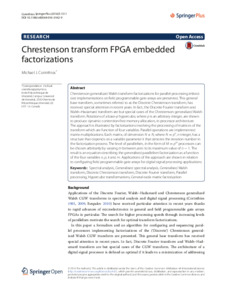Preview
Published Version
 Cite this document: Corinthios, M. J. (2016). Chrestenson transform FPGA embedded factorizations. SpringerPlus, 5(1), p. 1-23. doi:10.1186/s40064-016-3162-9

## Abstract

Chrestenson generalized Walsh transform factorizations for parallel processing imbedded implementations on field programmable gate arrays are presented. This general base transform, sometimes referred to as the Discrete Chrestenson transform, has received special attention in recent years. In fact, the Discrete Fourier transform and Walsh-Hadamard transform are but special cases of the Chrestenson generalized Walsh transform. Rotations of a base-p hypercube, where p is an arbitrary integer, are shown to produce dynamic contention-free memory allocation, in processor architecture. The approach is illustrated by factorizations involving the processing of matrices of the transform which are function of four variables. Parallel operations are implemented matrix multiplications. Each matrix, of dimension N x N, where N = p(n), n integer, has a structure that depends on a variable parameter k that denotes the iteration number in the factorization process. The level of parallelism, in the form of M = p(m) processors can be chosen arbitrarily by varying m between zero to its maximum value of n - 1. The result is an equation describing the generalised parallelism factorization as a function of the four variables n, p, k and m. Applications of the approach are shown in relation to configuring field programmable gate arrays for digital signal processing applications.

## Uncontrolled Keywords

Science; general; Spectral analysis; Generalised spectral analysis; Generalised Walsh transform; Discrete Chrestenson transform; Discrete Fourier transform; Parallel processing; Hypercube transformations; General-radix matrix factorization

 Subjects: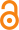Open Access document in PolyPublie 2500 Génie électrique et électronique > 2500 Génie électrique et électronique Département de génie électrique Non applicable CRSNG/NSERC 07 Dec 2018 13:26 08 Dec 2018 01:20 https://publications.polymtl.ca/3525/
 Journal Title: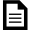Document issued by the official publisher SpringerPlus (vol. 5, no. 1) SpringerOpen https://doi.org/10.1186/s40064-016-3162-9